﻿ Speed Control of DC Motor

### Speed Control of DC Motor

Ľubica Miková, Ivan Virgala, Michal Kelemen

American Journal of Mechanical Engineering

## Speed Control of DC Motor

Ľubica Miková1,, Ivan Virgala1, Michal Kelemen1

1Department of Applied Mechanics and Mechatronics, Faculty of Mechanical Engineering, Technical University of Košice, Košice, Slovakia

### Abstract

The paper deals with design of control for DC motor and analysis with focus on speed control. Firstly, mathematical model of DC motor was created. For controller design two methods are used, namely frequency shaping method and PWM control. For creating and simulating the mathematical model Matlab program with Simulink extension was used. Both approaches are simulated and compared to each other. The contribution of paper brings information about advantages and disadvantages of approaches.

• Ľubica Miková, Ivan Virgala, Michal Kelemen. Speed Control of DC Motor. American Journal of Mechanical Engineering. Vol. 4, No. 7, 2016, pp 380-384. http://pubs.sciepub.com/ajme/4/7/27
• Miková, Ľubica, Ivan Virgala, and Michal Kelemen. "Speed Control of DC Motor." American Journal of Mechanical Engineering 4.7 (2016): 380-384.
• Miková, Ľ. , Virgala, I. , & Kelemen, M. (2016). Speed Control of DC Motor. American Journal of Mechanical Engineering, 4(7), 380-384.
• Miková, Ľubica, Ivan Virgala, and Michal Kelemen. "Speed Control of DC Motor." American Journal of Mechanical Engineering 4, no. 7 (2016): 380-384.

 Import into BibTeX Import into EndNote Import into RefMan Import into RefWorks

123
Prev Next

### 1. Introduction

Nowadays there are a lot of tasks in mechatronics which concern precise positioning in mechanisms. Mostly a friction phenomenon plays large problem in these tasks and many times it has to be compensated. [1, 2] DC motors find extensive applications in industries where precise speed control over a wider range of speed both above and below the rated speed.  Usually speed control of DC motor is achieved with PID controllers. The structure of the controller is generally obtained using conventional time/frequency domain analysis that includes Routh-Hurwitz criterion, pole placement technique, Root locus method, Ziegler-Nichols tuning formula etc. [4, 5] In our study two method of speed control will be investigated, namely frequency shaping method and PWM control.

The paper is divided into following sections: At first the mathematical model of a DC motor is introduced. Next, frequency shaping method is used for PD-controller and subsequently PWM controller is designed. Both controllers are compared to each other and results are discussed in the conclusion.

### 2. Mathematical Model of DC Motor

Mathematical model is described by means of differential equations which characterize the behaviour of analyzed system. For analyzing of system the mathematical model can be modelled in software and then it can be debugged. Then we can simulate the system and observe changing behaviour of system when we change the particular parameters of system. Mathematical model of DC motor consists of equations describing mechanical and electrical parts.Download asVeiw figureFigures index
Figure 1. Model of DC motor

Electrical part of DC motor can be described by following equations: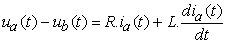(1)

Where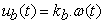(2)

Ia – current [A]

R – armature resistance [Ω]

L- armature inductance [H]

ua – supply voltage [V]

ub – induced voltage [V]

kb – electromotive force constant [Nms]

By Laplace transformation of equations (1) – (2) we obtain: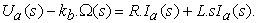(3)

Transfer function of equation (3) can be written as: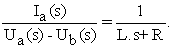(4)

Mechanical part of DC motor can be described by following equations: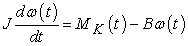(5)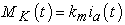(6)

where km is motor torque constant [Nm/A] and B is viscous friction coefficient. The transfer function of mechanical part of DC motor is: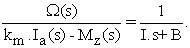(7)

The equation (7) can be expressed by block diagram, see Figure 2.Download asVeiw figureFigures index
Figure 2. Block diagram of DC motor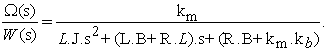### 3. Design of Controller for DC Motor Speed Control

3.1. Design of Controller by Frequency Response Shaping Method

Design of controller in frequency-domain is solved by frequency response shaping method of open loop system. Using this method the frequency response is shaped according to user requirements, namely in low-frequency domain, medium frequency domain and high-frequency domain. Modeling of log-magnitude frequency response of open loop system is a classic approach for designing of controller. It is a graphic method which is based on correcting of log-magnitude frequency response of open loop system so, that corrected frequency response suits to the requirements of control. Therefore it is necessary to design of controller C(s) in such a way that it would be able to stabilize the system and suits to following requirements of control quality:

In low-frequency domain – In the low-frequency domain the frequency response is designed according to requirements of precise control.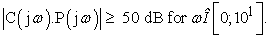In medium-frequency domain – The medium-frequency domain determines the quality of control, which by phase margin is characterized. The phase margin φm has to higher than 45°.

In high-frequency domain – The high-frequency domain does not have significant influence on quality of control but it has influence on magnitude of noise which is in this domain concentrated.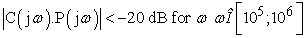In the Figure 3 Bode characteristics is shown.Download asVeiw figureFigures index
Figure 3. Bode characteristics of transfer function P(jω)

Bode characteristics of controlled system passes through restricted band in low-frequency domain and therefore it is necessary to use P-controller, which ensures the first requirement fulfillment. The gain of P-controller one obtain: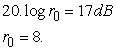(8)

Bode characteristic of controlled system using P-controller in the Figure 4 is shown.Download asVeiw figureFigures index
Figure 4. Result of using P-controller

From the Figure 4 can be seen, that phase margin is lower than 45° and it does not satisfies the second requirement for control quality. Therefore it is necessary to use PD-controller with gain r0=1 and break frequency ωD= 1000 rad/s.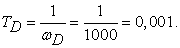(9)

The transfer function of controller in medium-frequency domain is now: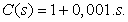(10)Download asVeiw figureFigures index
Figure 5. Bode characteristics using P and PD-controller

The Figure 5 shows that log-magnitude frequency response of open loop system 8. (1+0,001.s).P(s) passes through the restricted band in high-frequency domain. Therefore will be used low-pass filter with transfer function: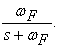(11)

In such way, that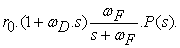(12)

It does not pass through the restricted band. In order to achieve this, it is necessary to choose the frequency ωF between values 104 - 105 rad/s. Transfer function of low-pass filter can be written as: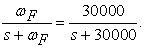(13)Download asVeiw figureFigures index
Figure 6. Final Bode characteristics of controlled system

The final transfer function of controller is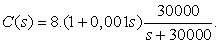(14)
3.2. DC Motor Speed Control by PWM Controller

In the next section, different way of controller design will be discussed. The pulse-width-modulation (PWM) has been widely used in the electronic and electrical systems including attitude control systems, adaptive control systems, signal processing, power control systems, and so forth. [6, 8] The reasons for the wide applicability of pulse-width-modulators are that the control variable has only two or three values such that the realization of PWM control is simple, and the pulse-width modulators can process large signals with high efficiency and low sensitivity to noise. Pulse-width modulation uses a square wave whose pulse width is modulated resulting in the variation of the average value of the waveform. If we consider a square waveform f(t) with a low value ymin, a high value ymax and a duty cycle D, the average value of the waveform is given by: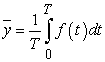(15)Download asVeiw figureFigures index
Figure 7. PWM pulse generate from comparing sinewave and sawtooth

As f(t) is a square wave, its value is ymax for 0<t<DT and ymin for DT<t<T. The above expression then becomes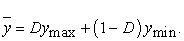(16)

This latter expression can be fairly simplified in many cases where ymin=0 as y=Dymax. From this, it is obvious that average value of the signal (y) is directly dependent on the duty cycle D.

The simplest way to generate a PWM signal is the intersective method, which requires only a sawtooth or a triangle waveform (easily generated using a simple oscillator) and a comparator. When the value of the reference signal (the green sine wave in figure 5) is more than the modulation waveform (blue), the PWM signal (magenta) is in the high state, otherwise it is in the low state. 

### 4. Simulation and Results

The aim of simulation is verification of designed controllers and comparison of them to each other. The simulations in Matlab / Simulink were done by creating the simulation scheme.

The step time of simulation is 0.0001 sec and as solver was used ode45 (Dormand-Prince).

The relative tolerance is set to 0.001. During the simulation, which lasts only one second, the reference signal changes every 0.35 seconds. For required speed of DC motor the block of random number is used with middle value 30 rad/sec. In the Figure 7 the final DC motor speed is shown.Download asVeiw figureFigures index
Figure 8. a) PD-controller in comparison with PWM controller b) Detail of course

As can be in the Figure 8 seen, there were used both controllers, PD and PWM as well. PD-controller follows the reference signal almost precise while PWM controller has a little bit oscillating course. For more precise PWM control it is necessary to use sawtooth signal with steeper slope, see Figure 7. In the simulation was also used saturation block for actuating signal limitation with value +- 10 volts.

### 5. Conclusion

In the paper DC motor speed control was investigated with focus on two methods, namely frequency response shaping method and PWM control. At first mathematical background of DC motor and then particular control methods were introduced. Then the simulation in Matlab / Simulink was done with solver ode45 (Dormand-Prince). The results shows that PD-controller with low-pass filter is more precise than PWM controller using our simulation conditions. So, by frequency shaping method can be designed the controller which suits required conditions of control.

### Acknowledgements

This contribution is also the result of the project implementation: Centre for research of control of technical, environmental and human risks for per-manent development of production and products in mechanical engineer-ing (ITMS:26220120060) supported by the Research & Development Operational Programme funded by the ERDF and VEGA 1/0872/16 Research of synthetic and biological inspired locomotion of mechatronic systems in rugged terrain.

### References

  T. Kara, I. Eker, “Nonlinear modeling and identification of a DC motor for bidirectional operation with real time experiments”, Energy and Management, Elsevier, 2003.In article  J. Horng, “Neural adaptive tracking control of a DC motor”, Informat Sci, 1999.In article  S. E. Lyshevski, Nonlinear control of mechatronic systems with permanent-magnet DC motors. Mechatronics, Vol. 9, pp 539-552, 1999.In article View Article  K. Sundareswaran, V. T. Sreedevi, DC Motor Speed Controller Design through a Colony of Honey Bees, Tencom 2008 IEEE Region 10 Conference, pp. 1-6.In article  B. C. Kuo, “Automatic Control Systems,” 7th edition, Prentice Hall of India, New Delhi, 1983.In article  Z. Liu, L. Jiang, PWM speed control system of DC motor based on AT89S51, International conference on electronic & mechanical engineering and information technology, 2011.In article  M. Farid, PID controller design for controlling DC motor speed using Matlab application, University Malaysia Pahang, 2008.In article  M. Kajan, L. Mrafko, F. Duchoň, P. Hubinský, J. Šovčfík :Control of automated guided vehicle with PLC SIMATIC ET200S CPU:, American Journal of Mechanical Engineering, Vol. 1, No. 7, 2013.In article